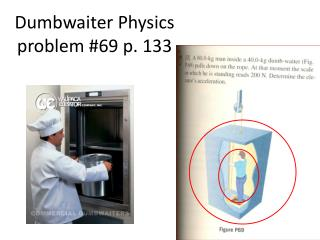DownloadDownload PresentationDumbwaiter Physics problem #69 p. 133

# Dumbwaiter Physics problem #69 p. 133

Download Presentation## Dumbwaiter Physics problem #69 p. 133

- - - - - - - - - - - - - - - - - - - - - - - - - - - E N D - - - - - - - - - - - - - - - - - - - - - - - - - - -
##### Presentation Transcript

1. Dumbwaiter Physicsproblem #69 p. 133

2. We will have 2 equations and 2 unknowns to solve simultaneously T T T N W elevator + man W man T + T – (M+m)g = (M + m) a T + N – mg = ma

3. Now plug in #s and simplify T + T – (M+m)g = (M + m) a T + N – mg = ma T + 200 – 80(9.8) = 80a 2T – (80+ 40)9.8 = (80+40) a T + 200 –784 = 80a 2T – 1176= 120 a T - 594 = 80a 2 equations with 2 unknowns: T and a. Solve one for T and plug into the other to eliminate the variable T. Then solve for a.